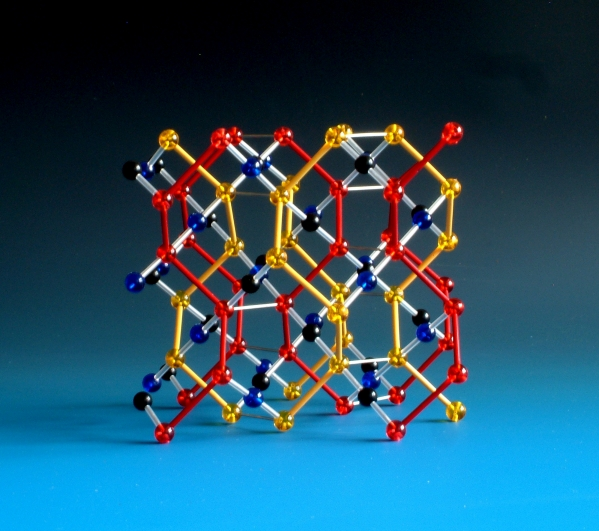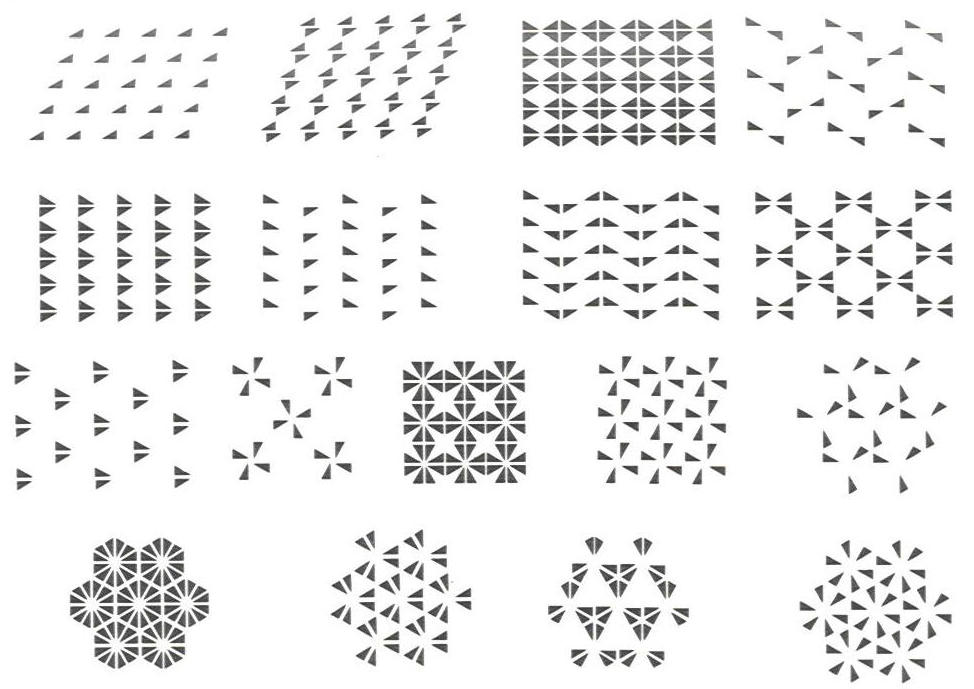Depiction of the Ia-3d space group

# Abstract depiction of space groups, plane groups and point groups

An understanding of space groups and the implications of space group symmetry on the physical and chemical properties of solids is central to many areas of structural science. At the same time, the symmetry of point groups, plane groups and space groups is not always the easiest of subjects to demonstrate to students.

The concept of the Bravais lattice is used to formally define a crystalline arrangement of points (e.g atoms or ions), but this alone is insufficient to reconstruct a crystal structure; we must also specify the internal symmetry of the unit cell (its point group) along with the lattice that the point groups sit within. This combination is known as a plane group (in the case of 2D lattices) or space group (for 3D lattices). There are 17 plane groups and 230 space groups in total.

## Point group molecular models

We can classify molecules according to their symmetry. The collection of symmetry elements present in a molecule forms a group, normally called a point group because all the symmetry elements (points, lines, and planes) of the molecule intersect at a single point. There are 32 distinct point groups in three dimensions, and most of these are represented by real molecules - only point group "O" has no real-world molecules and is instead represented by a conformation of a theoretical molecule.

## Plane groups

Asymmetric two-dimensional elements can be arranged in a regular, repeating patter in two dimensional arrays known as the plane groups. The plane groups provide a useful link between point group symmetry and space groups when teaching students about the latter. The primary symmetry elements of three dimensions are present, but by restricting the number of dimensions simplifies the possible combinations so that there are only 17 possible symmetrically distinct patterns. We have developed a set of models of these groups based around arrangements of an asymmetric motif of three atoms.

## Space groups

When it comes to space groups, we have yet to be asked to produce a set of all 230 space groups, but would be happy to take the challenge on if asked. We have, however, produced crystal structure models that are abstract forms of several space groups - they contain all the requisite symmetry elements of that space group, but with as little excess as possible to minimise confusion for the student. The model of space group Ia3d shown above is an excellent example of this approach - when compared with a real material with the same symmetry, such as garnet, the usefulness of an abstract depiction is immediately obvious.The 17 Plane groups